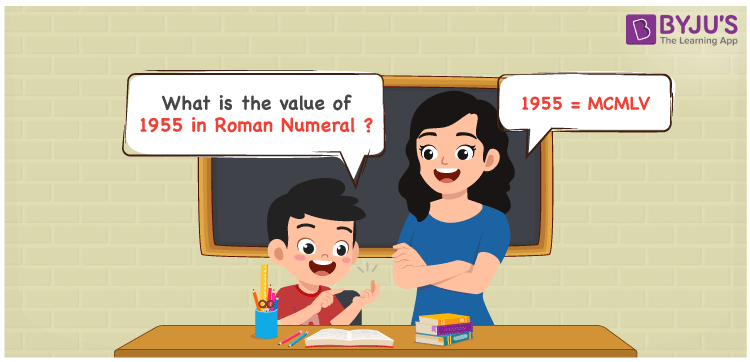# 1955 in Roman Numerals

1955 in Roman Numerals is MCMLV. Roman numerals is an additive and subtractive system where letters are used to show certain numbers in the number system. A different combination of symbols are used to indicate the roman numerals that include the English alphabet. Therefore, the number 1955 can be written as MCMLV in roman numerals.

 Number Roman Numeral 1955 MCMLV## How to Write 1955 in Roman Numerals?

In order to write 1955 in Roman Numerals, it has to be written in expanded form, i.e.

1955 = 1000 + 900 + 50 + 5

1955 = M + CM + L + V

1955 = MCMLV

## Video Lesson on Roman Numerals## Frequently Asked Questions on 1955 in Roman Numerals

Q1

### Write 1955 in Roman Numerals.

In Roman Numerals, 1955 is written as MCMLV.
Q2

### How to convert 1955 into Roman Numerals?

The conversion of 1955 into Roman Numerals includes breaking the number according to its place value.

Thousands = 1000 = M

Hundreds = 900 = CM

Tens = 50 = L

Ones = 5 = V

Number = 1000 + 900 + 50 + 5 = M + CM + L + V = MCMLV

Q3

### What Should be Added to 747 to Get 1955? Write the answer in Roman numerals.

1955 in roman numerals is MCMLV whereas 747 is DCCXLVII. 1955 – 747 = 1208. Therefore, 1208 should be added to 747 to get 1955. Now, to convert 1208 in roman numbers, we will express it in its expanded form, that is, 1208 = 1000 + 100 + 100 + 5 + 1 + 1 + 1 = M + C + C + V + I + I + I = MCCVIII.